Next: Predicted Orientation Contrast Up: Quantitative Comparisons with Previous: Quantitative Comparisons with

For the orientation adaptation to stimulus of angle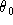, substituting equation (10) into equation (17), it is not difficult to derive that the network response to stimulus of angle 0 (vertical) is changed to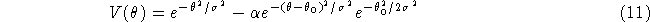in which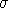is the feedforward tuning width chosen to be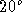and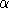is the parameter of the strength of decorrelation feedback.

The theoretical curve of perceived orientation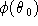is derived by assuming the maximum likelihood of the the neural population, i.e., the perceived angle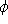is the angle at which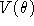is maximized. It is shown in figure 5 (right). The solid line is the theoretical curve and the experimental data come from  (they did not give the errors, the error bars are of our estimation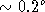). The parameter obtained through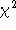fit is the strength of decorrelation feedback: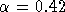.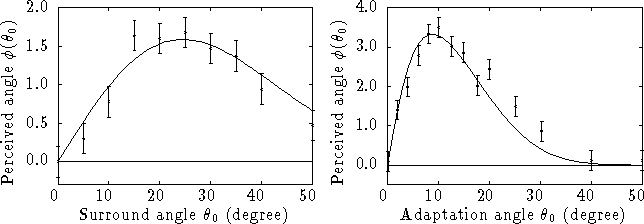Figure 5: Quantitative comparison of the theoretical predictions with the experimental data of orientation contrast (left) and orientation adaptation (right).

It is very interesting that we can derive a relationship which is independent of the parameter of the strength of decorrelation feedback,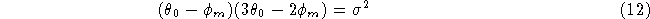in whichis the adaptation angle at which the tilt aftereffect is most significant and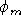is the perceived angle.Next: Predicted Orientation Contrast Up: Quantitative Comparisons with Previous: Quantitative Comparisons with